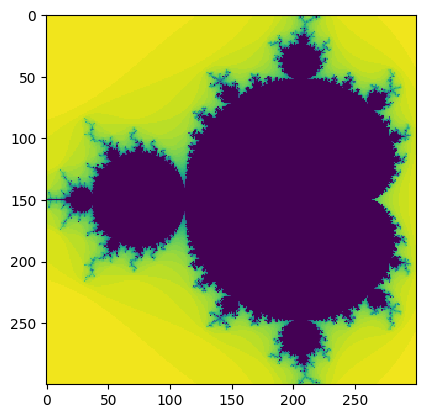Close

COMP0023: Research Software Engineering With Python

Home# Performance programming¶

We've spent most of this course looking at how to make code readable and reliable. For research work, it is often also important that code is efficient: that it does what it needs to do quickly.

It is very hard to work out beforehand whether code will be efficient or not: it is essential to Profile code, to measure its performance, to determine what aspects of it are slow.

When we looked at Functional programming, we claimed that code which is conceptualised in terms of actions on whole data-sets rather than individual elements is more efficient. Let's measure the performance of some different ways of implementing some code and see how they perform.

## Two Mandelbrots¶

You're probably familiar with a famous fractal called the Mandelbrot Set.

For a complex number $c$, $c$ is in the Mandelbrot set if the series $z_{i+1}=z_{i}^2+c$ (With $z_0=c$) stays close to $0$. Traditionally, we plot a color showing how many steps are needed for $\left|z_i\right|>2$, whereupon we are sure the series will diverge.

Here's a trivial python implementation:

In :
def mandel1(position, limit=50):

value = position

while abs(value) < 2:
limit -= 1
value = value**2 + position
if limit < 0:
return 0

return limit

In :
xmin = -1.5
ymin = -1.0
xmax = 0.5
ymax = 1.0
resolution = 300
xstep = (xmax - xmin) / resolution
ystep = (ymax - ymin) / resolution
xs = [(xmin + (xmax - xmin) * i / resolution) for i in range(resolution)]
ys = [(ymin + (ymax - ymin) * i / resolution) for i in range(resolution)]

In :
%%timeit
data = [[mandel1(complex(x, y)) for x in xs] for y in ys]

603 ms ± 3.03 ms per loop (mean ± std. dev. of 7 runs, 1 loop each)

In :
data1 = [[mandel1(complex(x, y)) for x in xs] for y in ys]

In :
%matplotlib inline
import matplotlib.pyplot as plt
plt.imshow(data1, interpolation='none')

Out:
<matplotlib.image.AxesImage at 0x7fa39d1960d0>We will learn this lesson how to make a version of this code which works Ten Times faster:

In :
import numpy as np
def mandel_numpy(position,limit=50):
value = position
diverged_at_count = np.zeros(position.shape)
while limit > 0:
limit -= 1
value = value**2+position
diverging = value * np.conj(value) > 4
first_diverged_this_time = np.logical_and(diverging, diverged_at_count == 0)
diverged_at_count[first_diverged_this_time] = limit
value[diverging] = 2

return diverged_at_count

In :
ymatrix, xmatrix = np.mgrid[ymin:ymax:ystep, xmin:xmax:xstep]

In :
values = xmatrix + 1j * ymatrix

In :
data_numpy = mandel_numpy(values)

In :
%matplotlib inline
import matplotlib.pyplot as plt
plt.imshow(data_numpy, interpolation='none')

Out:
<matplotlib.image.AxesImage at 0x7fa39d08efd0>In :
%%timeit
data_numpy = mandel_numpy(values)

40.4 ms ± 58.2 µs per loop (mean ± std. dev. of 7 runs, 10 loops each)


Note we get the same answer:

In :
sum(sum(abs(data_numpy - data1)))

Out:
0.0